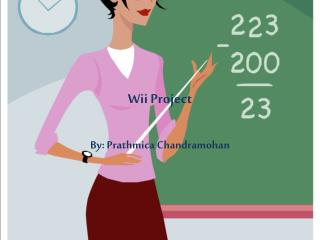DownloadDownload PresentationWii Project

# Wii Project

Download Presentation## Wii Project

- - - - - - - - - - - - - - - - - - - - - - - - - - - E N D - - - - - - - - - - - - - - - - - - - - - - - - - - -
##### Presentation Transcript

1. Wii Project By: Prathmica Chandramohan

2. Table

3. Table Contd…

4. Scatterplot

5. Trend of the Scatterplot • There is no trend to the scatterplot. • This is because there is not a pattern in the graph.

6. Independent and Dependent Variables • The independent variable are the players. • While the dependent variable is the amount of points the players get. • Remember that the y depends on x.

7. Domain • The Domain is the x value. • In this case, it is the number of players in the game.

8. Range • The range is the y value. • In this case, it is the number of points the players got.

9. Continuous or Discrete? • My graph is discrete because there is not a pattern to it.

10. Is it a Function? • It is a function because the x’s don’t repeat.

11. Compound Inequality for Domain

12. Compound Inequality for Range

13. The Average

14. Paragraph Describing the Average